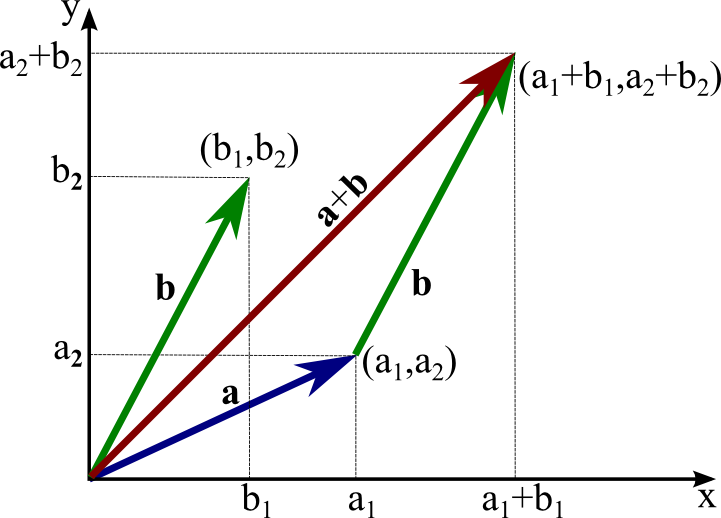# Math InsightA two-dimensional vector $\vc{b}=(b_1,b_2)$ is translated so that its tail is at the head of $\vc{a}=(a_1,a_2)$. The head of the sum $\vc{a}+\vc{b}$ is at the point $(a_1+b_1,a_2+b_2)$, illustrating how the coordinates of the sum are $\vc{a}+\vc{b} = (a_1+b_1,a_2+b_2)$.